Скачать презентацию Sampling Large Databases for Association Rules Jingting Zeng

5807f15acb40eebbb06c5c310edb6b09.ppt

• Количество слайдов: 26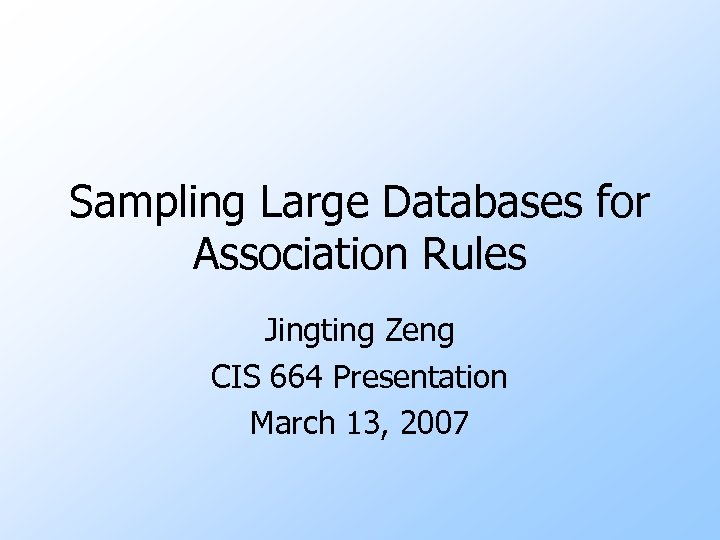Sampling Large Databases for Association Rules Jingting Zeng CIS 664 Presentation March 13, 2007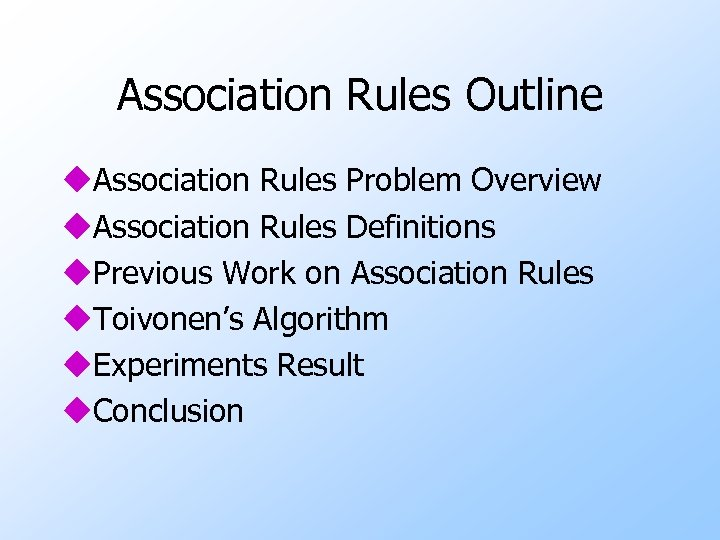Association Rules Outline u. Association Rules Problem Overview u. Association Rules Definitions u. Previous Work on Association Rules u. Toivonen’s Algorithm u. Experiments Result u. Conclusion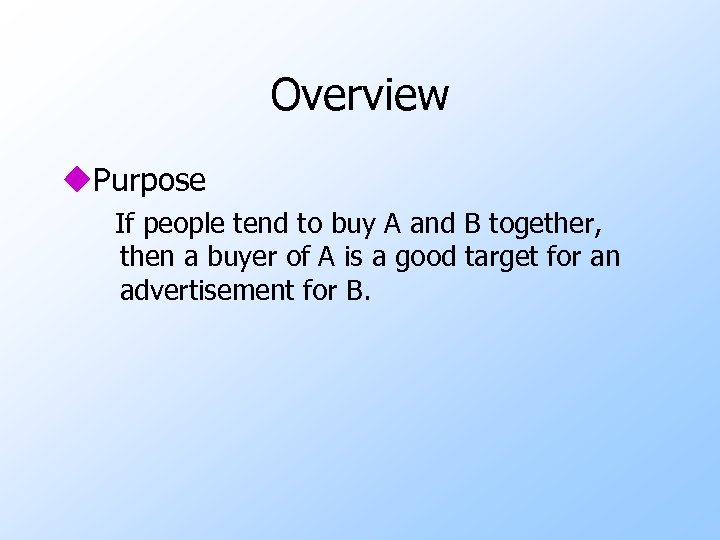Overview u. Purpose If people tend to buy A and B together, then a buyer of A is a good target for an advertisement for B.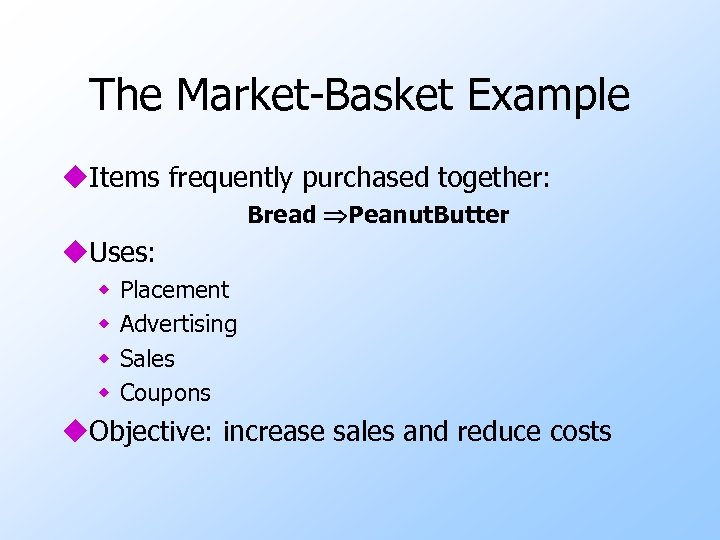The Market-Basket Example u. Items frequently purchased together: Bread Peanut. Butter u. Uses: w w Placement Advertising Sales Coupons u. Objective: increase sales and reduce costsOther Example u. The same technology has other uses University course enrollment data has been analyzed to find combinations of courses taken by the same students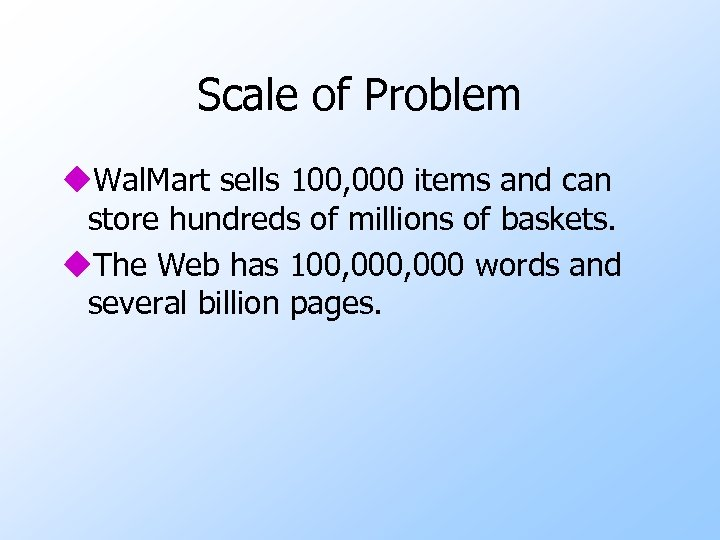Scale of Problem u. Wal. Mart sells 100, 000 items and can store hundreds of millions of baskets. u. The Web has 100, 000 words and several billion pages.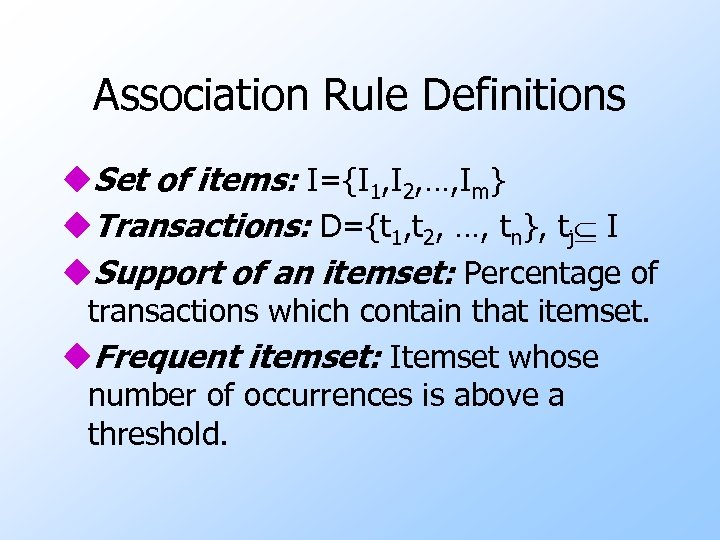Association Rule Definitions u. Set of items: I={I 1, I 2, …, Im} u. Transactions: D={t 1, t 2, …, tn}, tj I u. Support of an itemset: Percentage of transactions which contain that itemset. u. Frequent itemset: Itemset whose number of occurrences is above a threshold.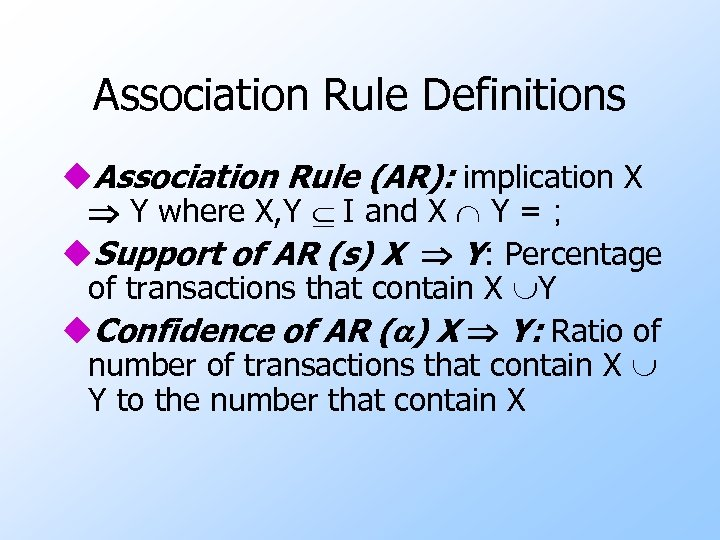Association Rule Definitions u. Association Rule (AR): implication X Y where X, Y I and X Y = ; u. Support of AR (s) X Y: Percentage of transactions that contain X Y u. Confidence of AR (a) X Y: Ratio of number of transactions that contain X Y to the number that contain X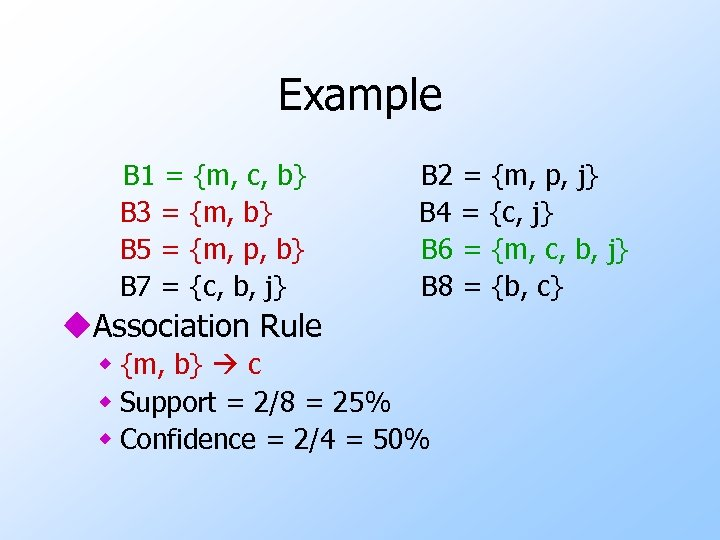Example B 1 = {m, c, b} B 3 = {m, b} B 5 = {m, p, b} B 7 = {c, b, j} B 2 = {m, p, j} B 4 = {c, j} B 6 = {m, c, b, j} B 8 = {b, c} u. Association Rule w {m, b} c w Support = 2/8 = 25% w Confidence = 2/4 = 50%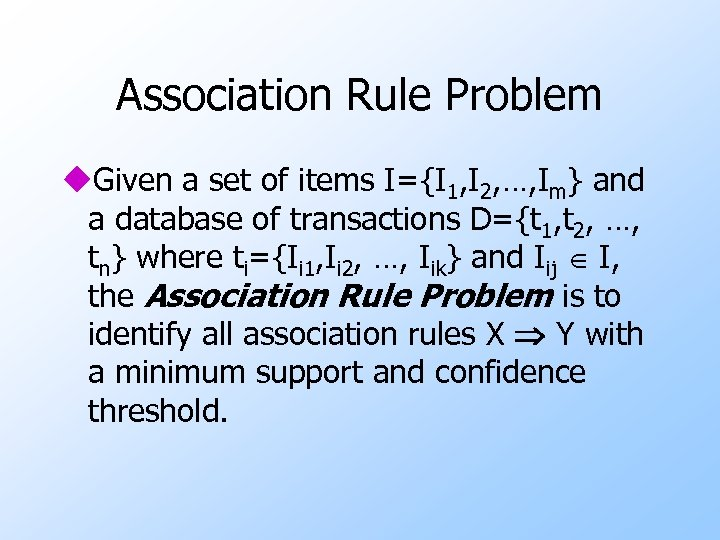Association Rule Problem u. Given a set of items I={I 1, I 2, …, Im} and a database of transactions D={t 1, t 2, …, tn} where ti={Ii 1, Ii 2, …, Iik} and Iij I, the Association Rule Problem is to identify all association rules X Y with a minimum support and confidence threshold.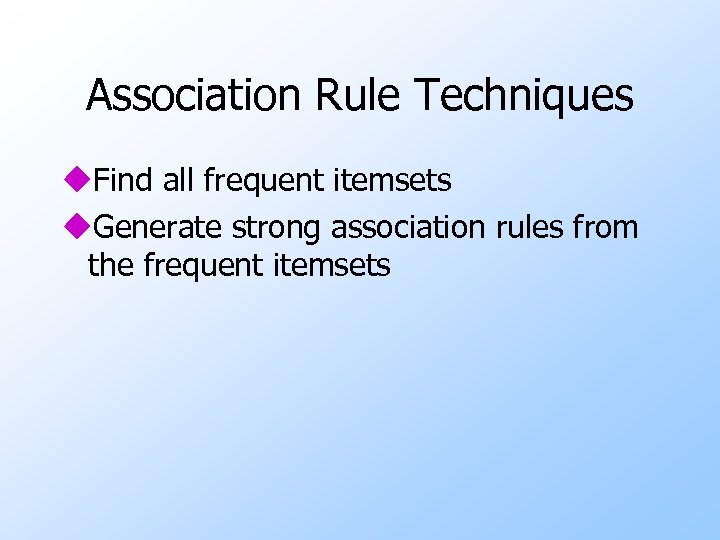Association Rule Techniques u. Find all frequent itemsets u. Generate strong association rules from the frequent itemsets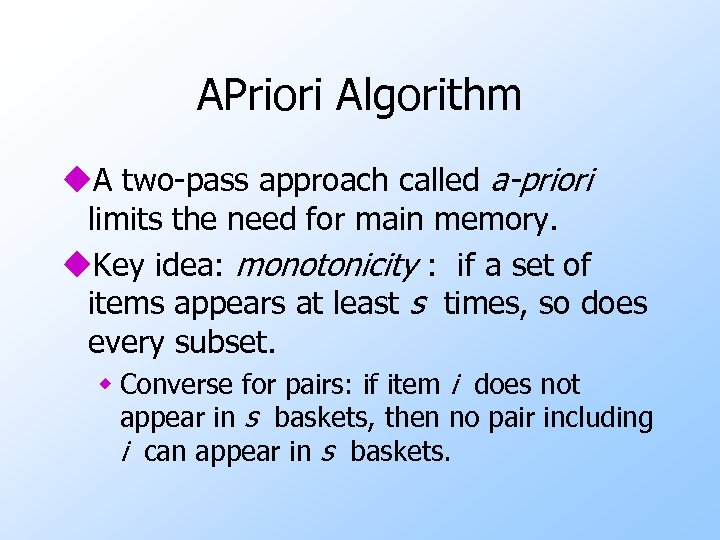APriori Algorithm u. A two-pass approach called a-priori limits the need for main memory. u. Key idea: monotonicity : if a set of items appears at least s times, so does every subset. w Converse for pairs: if item i does not appear in s baskets, then no pair including i can appear in s baskets.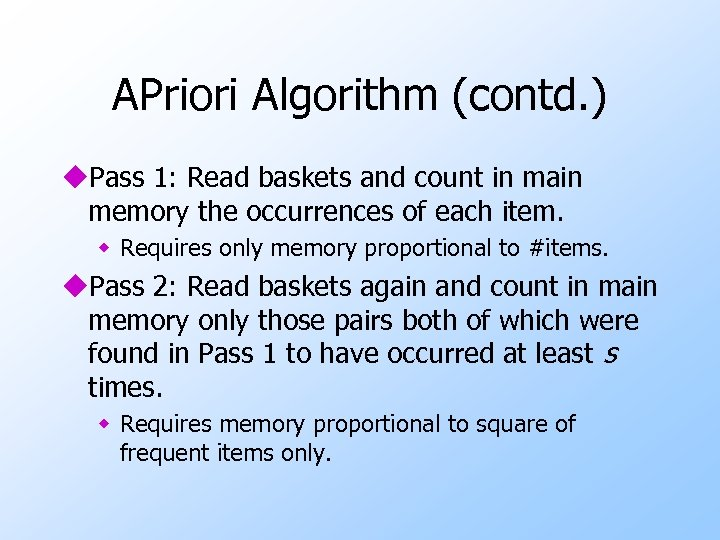APriori Algorithm (contd. ) u. Pass 1: Read baskets and count in main memory the occurrences of each item. w Requires only memory proportional to #items. u. Pass 2: Read baskets again and count in main memory only those pairs both of which were found in Pass 1 to have occurred at least s times. w Requires memory proportional to square of frequent items only.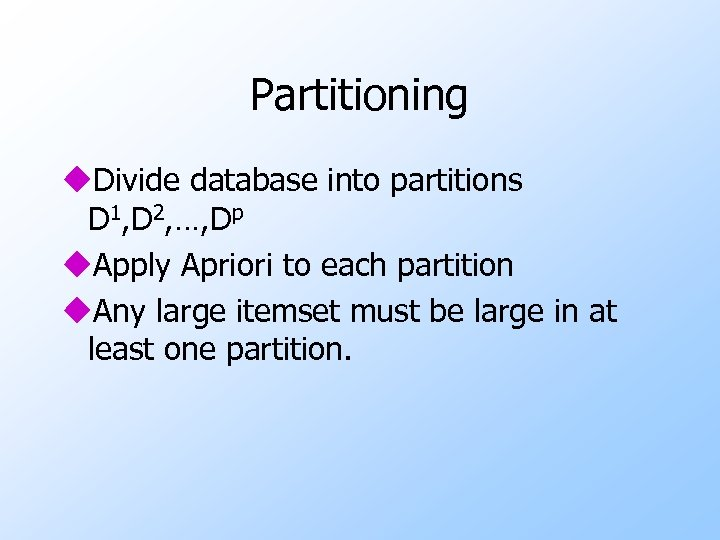Partitioning u. Divide database into partitions D 1, D 2, …, Dp u. Apply Apriori to each partition u. Any large itemset must be large in at least one partition.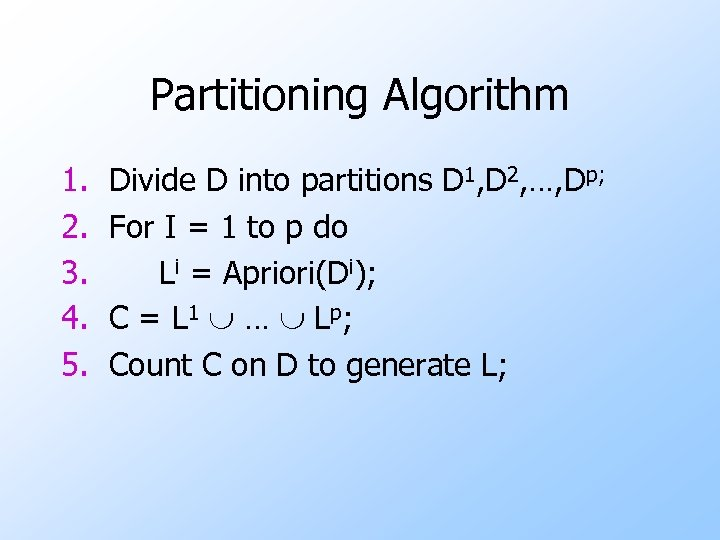Partitioning Algorithm 1. 2. 3. 4. 5. Divide D into partitions D 1, D 2, …, Dp; For I = 1 to p do Li = Apriori(Di); C = L 1 … Lp; Count C on D to generate L;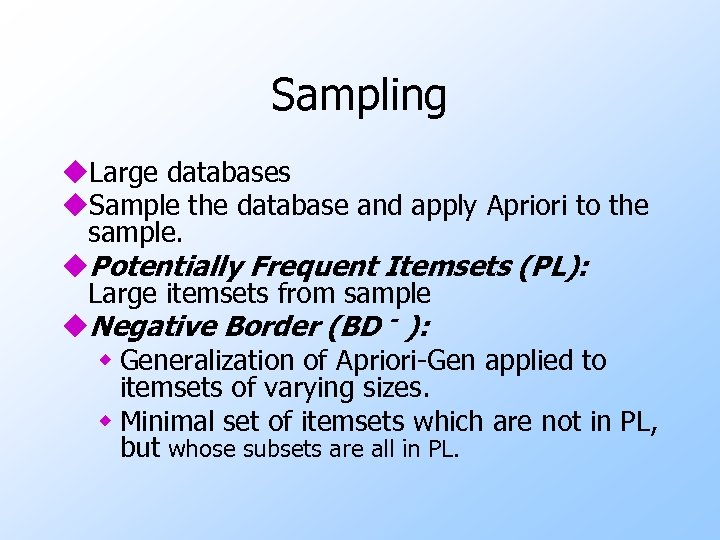Sampling u. Large databases u. Sample the database and apply Apriori to the sample. u. Potentially Frequent Itemsets (PL): Large itemsets from sample u. Negative Border (BD - ): w Generalization of Apriori-Gen applied to itemsets of varying sizes. w Minimal set of itemsets which are not in PL, but whose subsets are all in PL.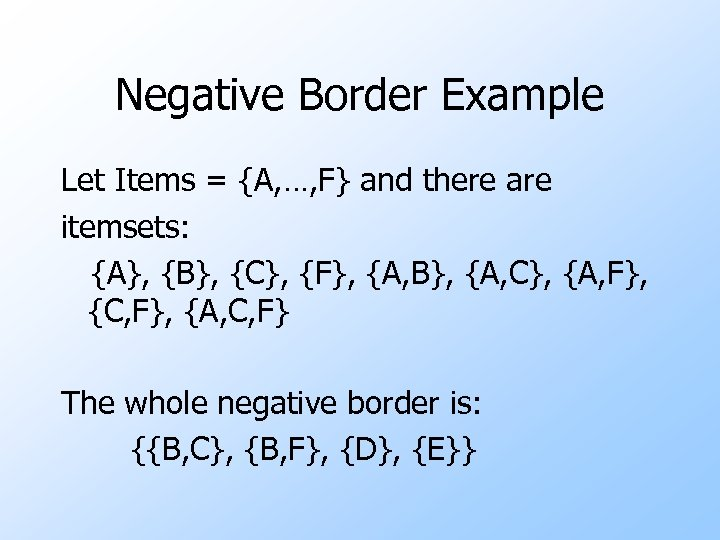Negative Border Example Let Items = {A, …, F} and there are itemsets: {A}, {B}, {C}, {F}, {A, B}, {A, C}, {A, F}, {C, F}, {A, C, F} The whole negative border is: {{B, C}, {B, F}, {D}, {E}}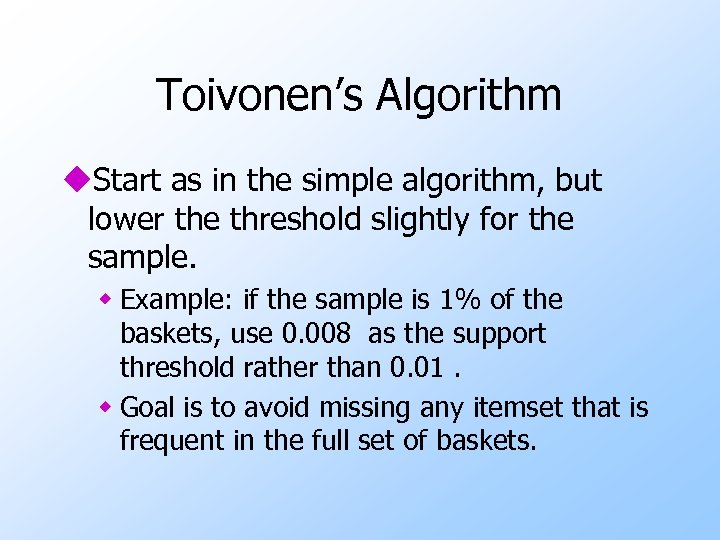Toivonen’s Algorithm u. Start as in the simple algorithm, but lower the threshold slightly for the sample. w Example: if the sample is 1% of the baskets, use 0. 008 as the support threshold rather than 0. 01. w Goal is to avoid missing any itemset that is frequent in the full set of baskets.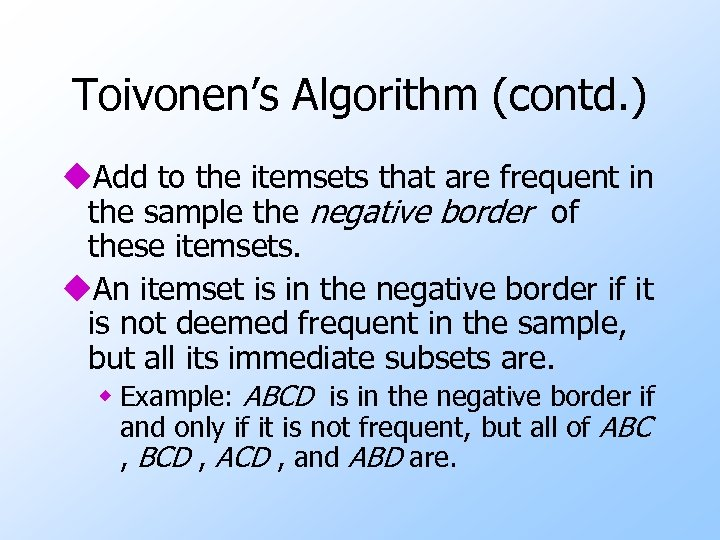Toivonen’s Algorithm (contd. ) u. Add to the itemsets that are frequent in the sample the negative border of these itemsets. u. An itemset is in the negative border if it is not deemed frequent in the sample, but all its immediate subsets are. w Example: ABCD is in the negative border if and only if it is not frequent, but all of ABC , BCD , ACD , and ABD are.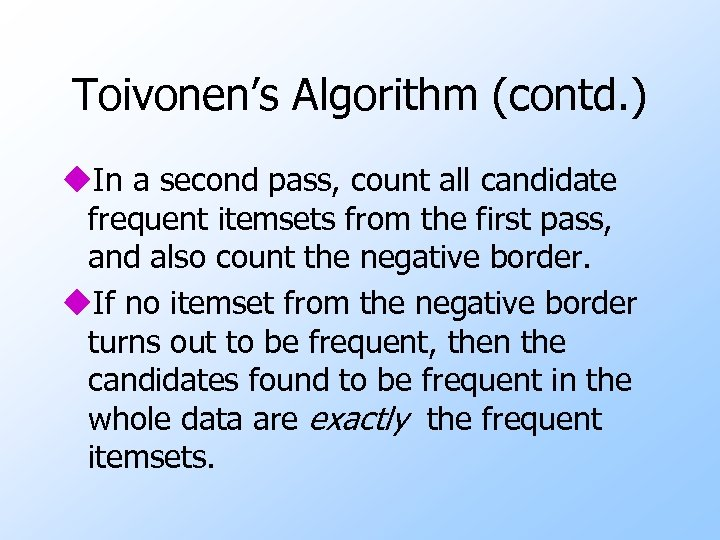Toivonen’s Algorithm (contd. ) u. In a second pass, count all candidate frequent itemsets from the first pass, and also count the negative border. u. If no itemset from the negative border turns out to be frequent, then the candidates found to be frequent in the whole data are exactly the frequent itemsets.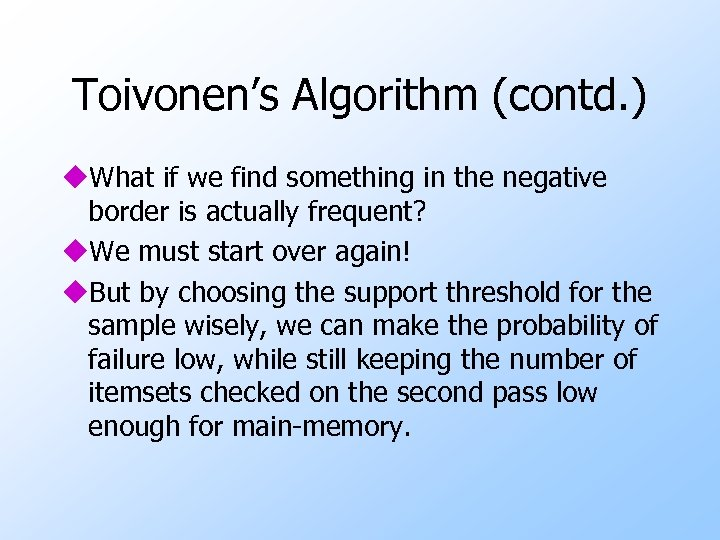Toivonen’s Algorithm (contd. ) u. What if we find something in the negative border is actually frequent? u. We must start over again! u. But by choosing the support threshold for the sample wisely, we can make the probability of failure low, while still keeping the number of itemsets checked on the second pass low enough for main-memory.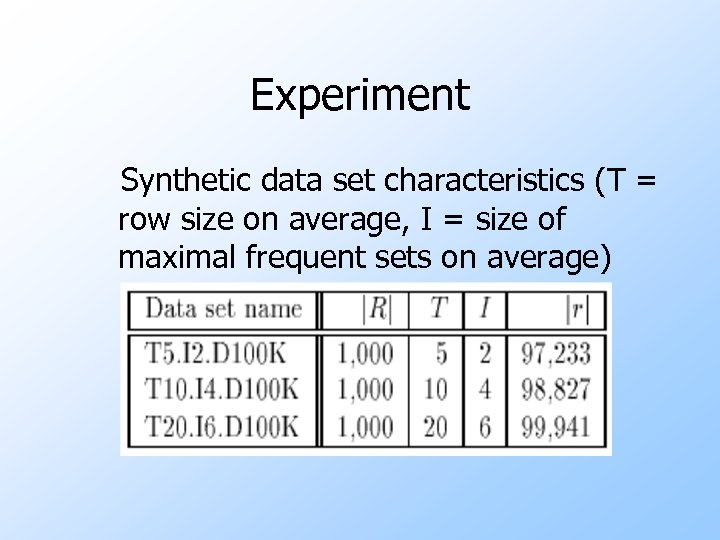Experiment Synthetic data set characteristics (T = row size on average, I = size of maximal frequent sets on average)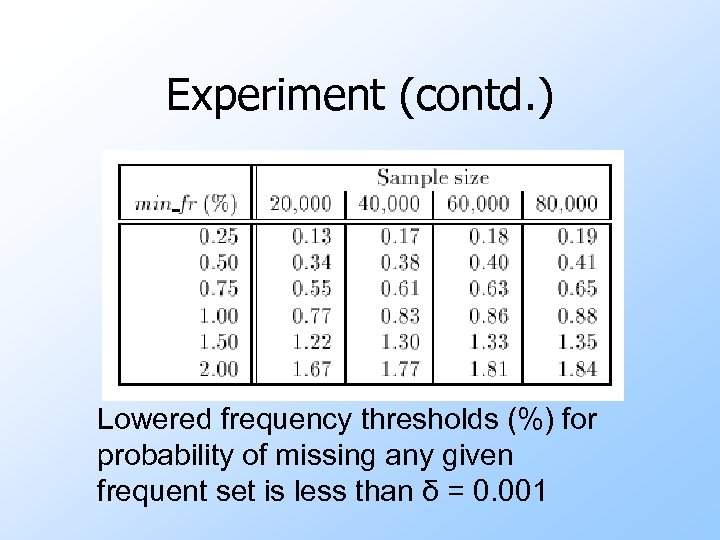Experiment (contd. ) Lowered frequency thresholds (%) for probability of missing any given frequent set is less than δ = 0. 001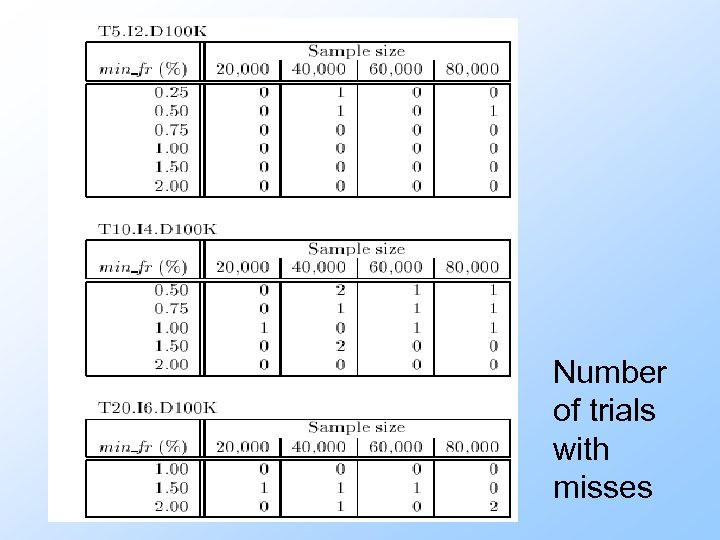Number of trials with misses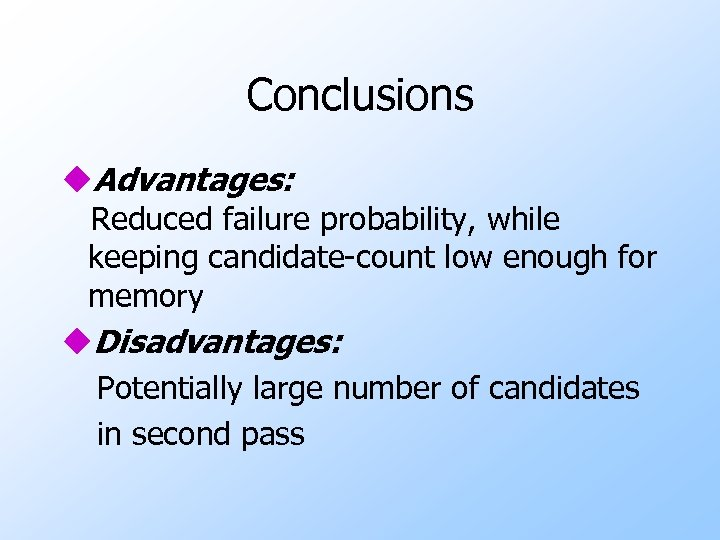Conclusions u. Advantages: Reduced failure probability, while keeping candidate-count low enough for memory u. Disadvantages: Potentially large number of candidates in second passThank you!